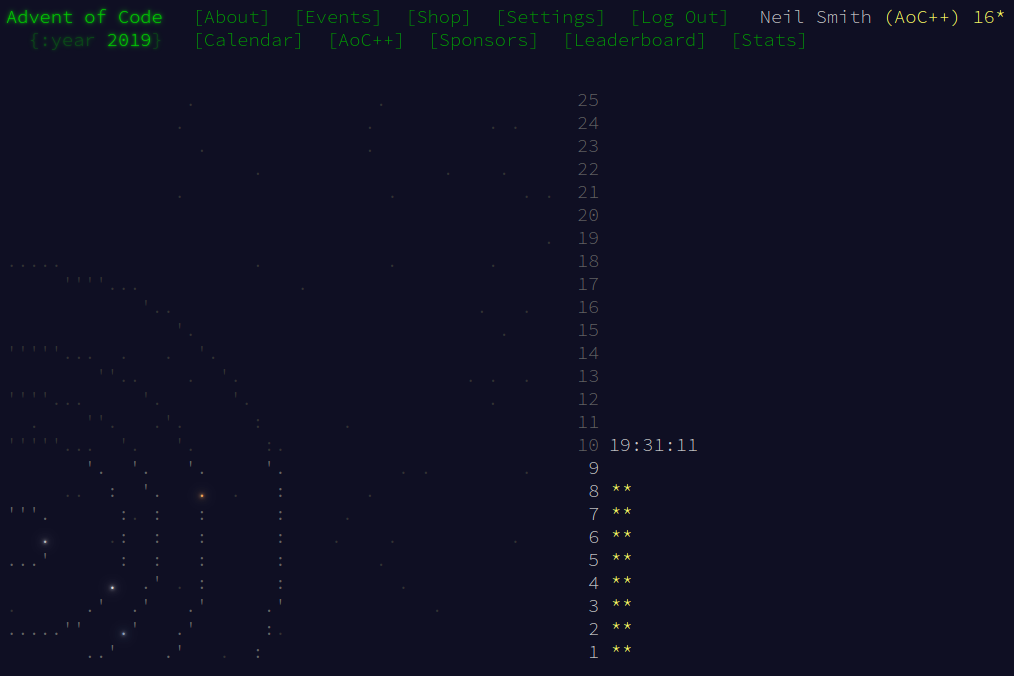December 9, 2019

# Advent of Code 2019 day 8

Some gentle data reshapingAfter the complexity of yesterday, Day 8 was some light relief. It also uses quite a few of Haskell's generic data manipulation functions from the standard library.

For once, I didn't use Megaparsec for parsing the data file: just read the file and map digitToInt over it to convert the characters into digits. The built-in chunksOf splits the raw string into layers.

Part 1 becomes clear through the use of the count utility function, and the built-in comparing function to find the layer with the most zeroes.

In part 2, I use transpose to convert the data from being a layer upon layer of pixels, to being a list of "pixels", but each "pixel" is in fact the value of that pixel in all the layers in the image. I can find the colour of each pixel by dropping all the leading transparent pixels and picking the first of what's left.

I then convert these visible pixels into the strings that will represent them on the screen. concatMap does the conversion and represents the image as a single one-dimensional string; the chunksOf / unlines pair reshapes that row into the grid.

## Code

Here's the entirety of the code (and Github).

import Data.List
import Data.List.Split
import Data.Char
import Data.Ord

main :: IO ()
main = do
let digits = successfulParse text
let layers = chunksOf (imageWidth * imageHeight) digits
print $part1 layers putStrLn$ part2 layers

imageWidth = 25
imageHeight = 6

part1 layers = (count 1 target) * (count 2 target)
where target = minimumBy (comparing (count 0)) layers

part2 layers = unlines rows
where pixelLayers = transpose layers
pixels = map firstVisible pixelLayers
image = concatMap showPixel pixels
rows = chunksOf imageWidth image

firstVisible = head . dropWhile (== 2)

showPixel 0 = " "
showPixel 1 = "\x2588"

count n = length . filter (== n)

successfulParse :: String -> [Int]
successfulParse input = map digitToInt input